## Monochromatic Energy for Low Energy Bands

### Introduction

As discussed in the Why topic: Selecting a Monochromatic Energy, there are several approaches to selecting a monochromatic energy appropriate for any arbitrary energy band. This page extends that topic and discusses the challenges when selecting a monochromatic energy for soft energy bands (low energies).

The Chandra Source Catalog establishes a soft-energy band that goes from 0.5-1.2 keV. The same energy limits are used for this analysis. The CSC project also adopted a value of 0.92keV as the characteristic monochromatic energy for this band. The CSC uses this value is used as the characteristic monochromatic energy creating exposure maps. The exposure maps are then used when computing photon fluxes (photflux_aper_s). The srcflux script uses the exposure map in the same way to compute net_photflux_aper values. These photon fluxes and exposure maps are also used when computing certain hardness ratio values and when computing limiting sensitivity. In addition, this value is used in release 1 of the CSC for creating monochromatic PSFs.

### Why is the soft band special?

First, the ACIS effective area is variable due to the continued build up of a spatially varying contaminant on the detector. This affects the detector quantum efficiency predominantly at low energies (generally less than 1keV). In Figure 1. we show the effective area for a source at the aimpoint on the ACIS-I detector. Each curve is for a different observation cycle ("ao") -- basically a separate curve for each calendar year.

### Figure 1. ACIS-I response vs. Observation Date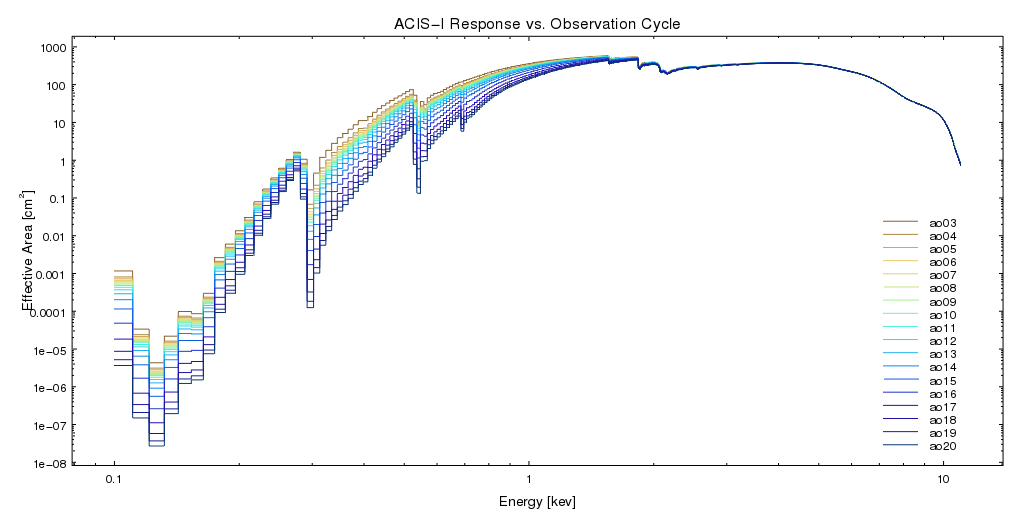### Figure 1. ACIS-I response vs. Observation Date

In this figure we plot the ARF for the ACIS-I aimpoint. These ARF files are created at essentially the same location on the detector and for the same time as the PIMMS Effective Area curves (from cycle 3 through 20). These ARF files have all been remade using CIAO 4.10 with CALDB 4.7.9. For a discussion of why the canned PIMMS effective area curves should not be use see the Pitfalls using PIMMS for Observed Data page. Note that the AO20 data point represents the predicted response in the middle of AO20, ie May 2019 (extrapolated from data available in 2018).

The response above ~1.4 keV is nearly constant. However, the soft band response varies considerably with time.Note regarding Chandra Source Catalog sources

For reference, CSC 1.1 included data publicly available through 2009, corresponding to AO10. CSC 2.0 includes data publicly available through 2014, corresponding to AO15.

Second, the intrinsic quantum efficiency of the ACIS CCDs in the soft band rapidly increases with increasing energy, peaking at the 1.49keV Si edge where the efficiency drops.

Considering these two effects, we then see that any spectral model, folded through the response at the same location on the detector and imaged at the same off-axis angle will produce a different spectral weighted mean energy depending on the date of the observation. We can see this in Figure 2.

### Figure 2. Mean Energy for Absorbed Power law in Soft Band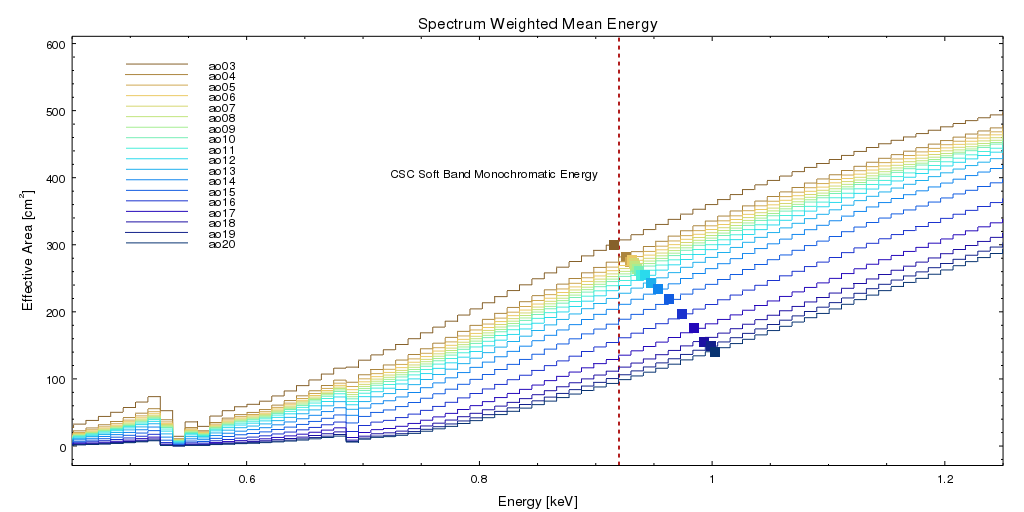### Figure 2. Mean Energy for Absorbed Power law in Soft Band

Same ARFs as in Figure 1, zoomed into the CSC soft band, showing the spectral weighted mean energy based on an absorbed power law with nH=0.03 and PhoIndex=1.7.

The vertical dashed line at 0.92keV is the standard CSC soft-band monochromatic energy.

The solid square symbol shows the spectral weighted mean energy for each year using the same spectral model.

### What happens when we use a fixed monochromatic energy?

We can replot the data in Figure 2 to show the spectral weighted mean energy versus time. This is shown in Figure 3.

### Figure 3. Spectral Weighted Mean Energy vs. Observation cycle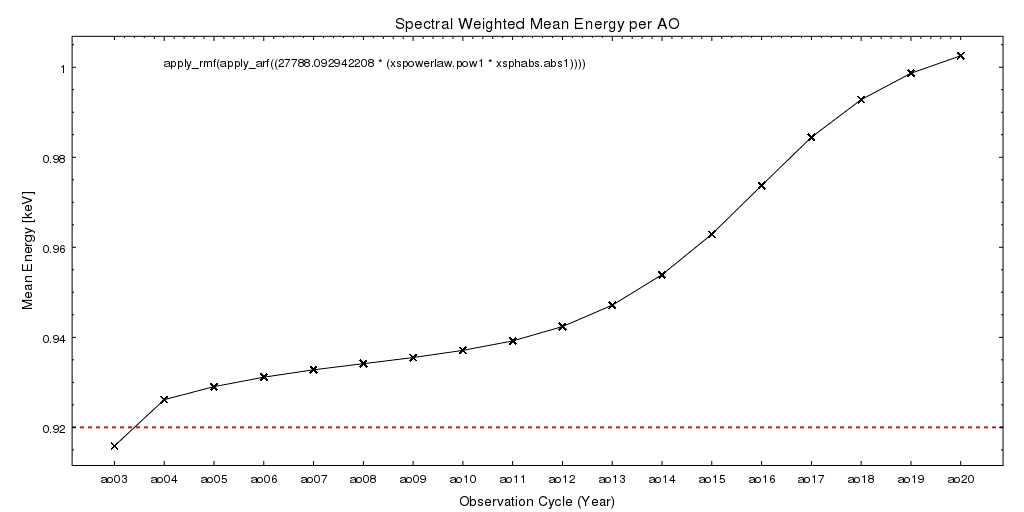### Figure 3. Spectral Weighted Mean Energy vs. Observation cycle

This plot shows the same same information as in Figure 2 with the observation date (AO) on the X-axis and the mean energy for our canonical absorbed powerlaw folded through the ARF file for that time period. The mean energy is computed in the CSC soft band (0.5 to 1.2keV); the standard CSC monochromatic energy for the soft band is 0.92keV and is shown as the dashed horizontal line.

We see in Figure 3 that the spectral weighted mean energy, assuming a fixed spectral model, changes over the lifetime of the mission. However, the change appears relatively small, on the order of only 80eV. The more interesting question is how much does the effective area change? Specifically, how much does the effective area at 0.92keV compare to the effective area at the spectral weighted mean energy? This is shown in Figure 4.

### Figure 4. Percent Difference in Effective Area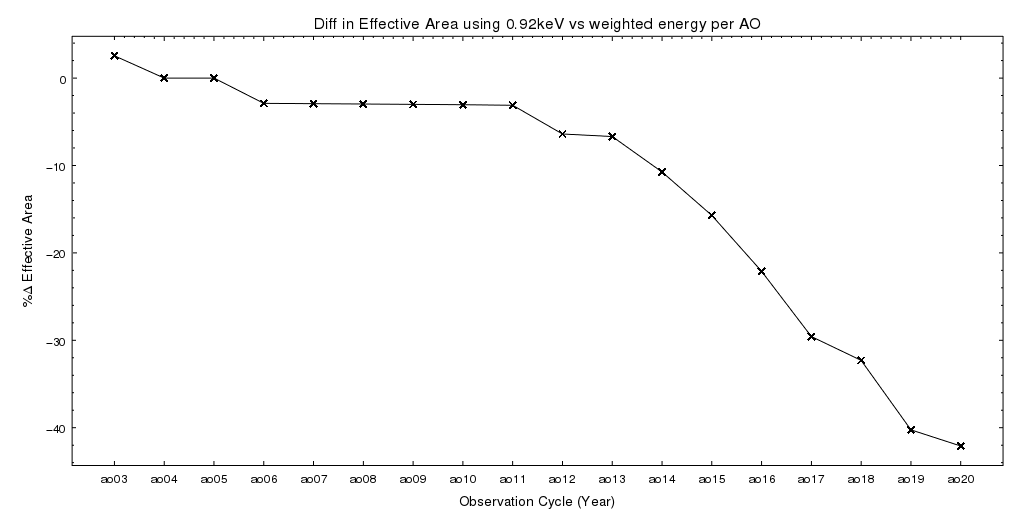### Figure 4. Percent Difference in Effective Area

The percent difference in the effective area at 0.92keV compared to the effective area at the spectral weighted mean energy in the CSC soft band using the standard absorbed powerlaw model folded through the ARF file for each observation cycle.

Figure 4 shows that even though the change in mean energy is small (only about 80eV), because the slope of the effective area curve is very steep in the soft band, a small change in energy results in a large change in effective area. For the standard absorbed power law model used here the difference in effective area is over 40% in AO20 which will result in photon flux estimates being 1.67 times higher than in early AOs. So, even though the count rate will be lower in AO20 compared to AO03, the photon flux will be higher.

Another way to think about this is to go back and revisit the canonical absorbed power law model. With the early mission effective area curves using an absorbed powerlaw with nH=0.03 and PhoIndex=1.7 we obtain a spectral weighted mean energy of 0.92keV. If we use the late mission effective area curves, what model parameters are needed to produce the same mean energy?

### Figure 5. Varying Absorbed Power Law Model Parameters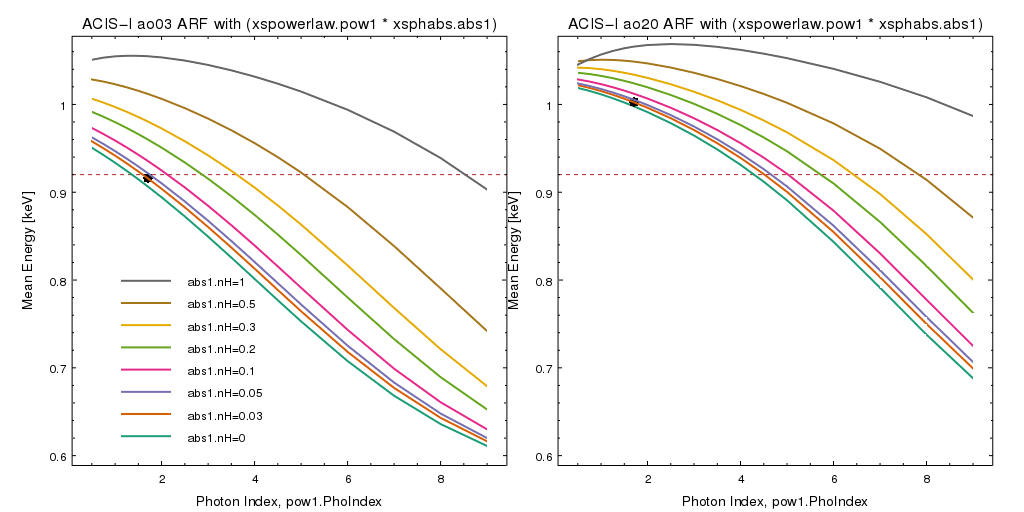### Figure 5. Varying Absorbed Power Law Model Parameters

The spectral weighted mean energy obtained by varying the parameters of the absorbed power law model. (Left) includes the ARF file for AO03, (Right) includes the ARF file for AO20. The curves are for fixed values of nH.

The black circle shows the mean energy for the standard nH=0.03 with PhoIndex=1.7.

In Figure 5 we show how the computed mean energy varies with model parameters if we use the early AO03 ARF vs. the later AO20 ARF. The horizontal dashed line shows the nominal 0.92keV monochromatic energy. For the early AO data (left) we can see that there is a large range of nH and PhoIndex values which yield a mean energy around 0.92 keV. However, the later AO data (right) shows that with nH=0, we cannot get a mean energy equal to 0.92keV until the photon index of the power law model component is over 4.

Therefore, another way to think of this effect is that by adopting a static monochromatic value we are in essence choosing to vary the spectral model parameters with time.

This study was initiated based on the observation that three-color images using the standard CSC energy band definitions for recently obtained datasets appeared to all systematically have a larger soft-band contribution. In simple terms: the images appeared more red. We see this in Figure 6 where the calibration target, Abell1795, appears to have an overall increase in soft-band photon flux causing the three-color image to have a red hue.

### Figure 6. Three-Color Images of Calibration Target Abell 1795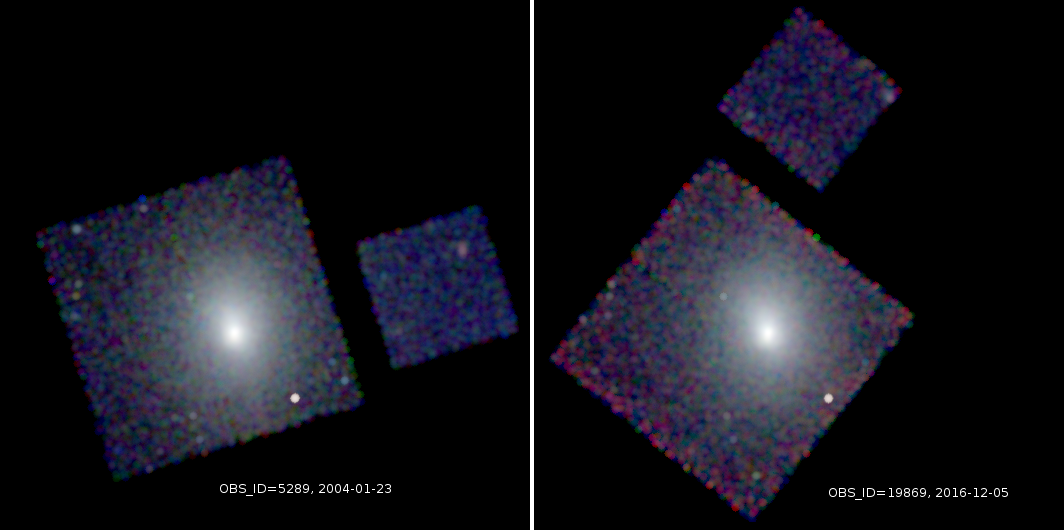### Figure 6. Three-Color Images of Calibration Target Abell 1795

Three-color images of the Abell1795 cluster used in the Chandra calibration program. Red corresponds to the CSC soft-band, green to the CSC medium band, and blue to the CSC hard band. The images have been smoothed and exposure corrected using exposure maps created at the standard CSC monochromatic energies for each band.

(Left) is OBS_ID 5289 taken on 2004-01-23. (Right) is OBS_ID 19869 taken on 2016-12-06. The relative increase in the soft-band photon flux is evidenced by the increase in the red-channel of the later dataset especially visible around the edge of the detector.

This is of course counter intuitive. Given the increase in contamination we might expect the soft-band flux to decrease with time; not increase.

What is happening is that the soft band counts are indeed decreasing; but the mean energy of those events that are detected is increasing. By choosing a fixed monochromatic energy those counts are being corrected for a lower effective area ; thus causing the flux to be biased higher.

This figures shows the extremes of a very early to a very recent dataset; there are many additional observations during the mission which show how the soft-band changes incrementally. Obviously other variables such as changes in the background and other factors may also contribute to this change; however, this effect is seen in all the more recent datasets so it suggests something more systematic which is what lead this analysis.

### So then is using 0.92keV wrong?

No.

The choice of 0.92keV is fine. From this analysis it is clear that any fixed monochromatic energy used for the soft-band will be subject to the same biases. This is due to the steep slope of the effective area curve in this part of the spectrum. In the other energy bands the effective area changes more gradually with energy so they are less sensitive to small energy changes.

Care must be taken when interpreting the soft band photon flux values. The values are: the soft band counts divided by the effective area at 0.92keV. That is all that can be said. It would be wrong to suggest that 0.92keV represents the spectral weighted mean energy for our standard absorbed power law -- it may for some observations, but may not for others.

Users should also be cautious when comparing soft band photon fluxes in the context of long-term temporal variability.